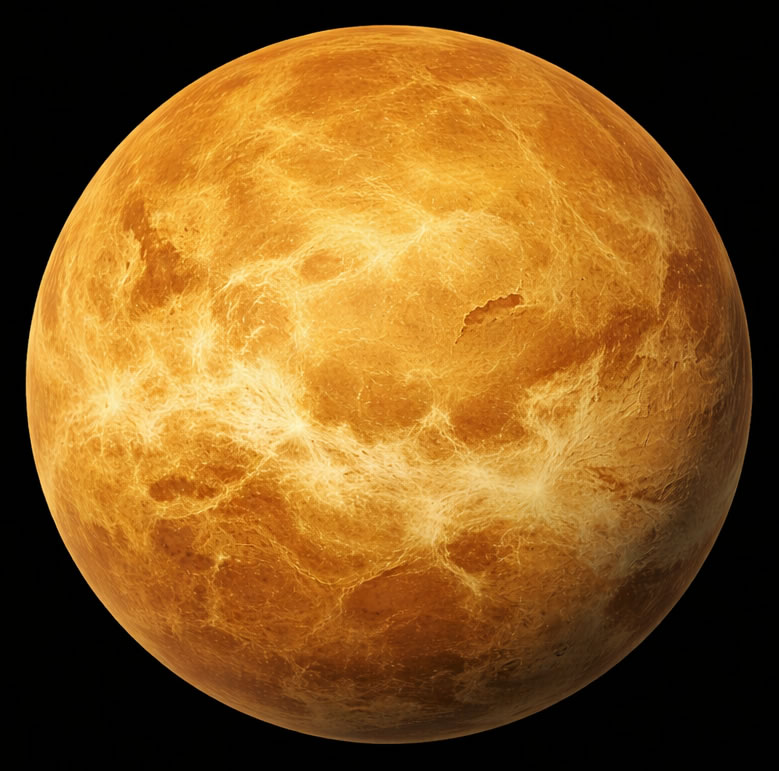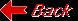Planet VenusMass (kg) 4.869e+24 Mass (Earth = 1) .81476 Equatorial radius (km) 6,051.8 Equatorial radius (Earth = 1) .94886 Mean density (gm/cm^3) 5.25 Mean distance from the Sun (km) 108,200,000 Mean distance from the Sun (Earth = 1) 0.7233 Rotational period (days) -243.0187 Orbital period (days) 224.701 Mean orbital velocity (km/sec) 35.02 Orbital eccentricity 0.0068 Tilt of axis (degrees) 177.36 Orbital inclination (degrees) 3.394 Equatorial surface gravity (m/sec^2) 8.87 Mean surface temperature 482C Atmospheric composition: Carbon dioxide, Nitrogen Trace amounts of: Sulfur dioxide, water vapor, carbon monoxide, argon, helium, neon, hydrogen chloride, and hydrogen fluoride. 96%, 3+%